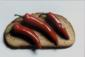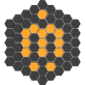# Modification on this script... 3d ui align set a minimum handle length

I tried contacting the creator but no luck.

The script works great, but there is one annoying thing, the handle length is based on the selection of the verts. So if you have verts that are just slightly off and you run the script you have to zoom in a lot to see which handle alignment you are activating.

Basically i would like the handles to have a set minimum length so they are easy to see and select.

Anyone out there that could modify it to work, would be much appreciated.

thx

here is the script:

--------------------------------------
-- 3D UI Vertex Align Tool v1.0 --
-- Created by Trent Fealy --
-- www.studiosyntax.net --
--------------------------------------

global VertexAligner
global draw = false

global SelectedPoint = 0
global X = #([0,0,0], [0,0,0], [0,0,0])
global Y = #([0,0,0], [0,0,0], [0,0,0])
global Z = #([0,0,0], [0,0,0], [0,0,0])

global P = #([0,0,0], [0,0,0])

global screenpoints= #([0,0,0], [0,0,0], [0,0,0], [0,0,0], [0,0,0], [0,0,0], [0,0,0])

global BitVertArray
global VertArray
global amount

fn Align =
(

selectionarray = getCurrentSelection()

if classOf selectionarray == Editable_Poly do
(
BitVertArray = polyop.getVertSelection selectionarray
VertArray = BitVertArray as array
amount = BitVertArray.count
print "asda"
for i = 1 to amount do
(
if BitVertArray[i] == true do
(
original = polyop.getVert selectionarray i

if SelectedPoint == 1 do
(
original=X
)

if SelectedPoint == 2 do
(
original=X
)

if SelectedPoint == 3 do
(
original=Y
)

if SelectedPoint == 4 do
(
original=Y
)

if SelectedPoint == 5 do
(
original=Z
)

if SelectedPoint == 6 do
(
original=Z
)

polyop.setVert selectionarray i original

)

)

)
)

fn AlignInfo =
(
selectionarray = getCurrentSelection()

if classOf selectionarray == Editable_Poly do
(
BitVertArray = polyop.getVertSelection selectionarray
VertArray = BitVertArray as array
amount = BitVertArray.count

init = false

for i = 1 to amount do
(
if BitVertArray[i] == true do
(
original = polyop.getVert selectionarray i

if init == false do
(
X= original
X= original

Y= original
Y= original

Z= original
Z= original
)

init = true

if original < X do
(
X = original
)
if original > X do
(
X = original
)

if original < Y do
(
Y = original
)
if original > Y do
(
Y = original
)

if original < Z do
(
Z = original
)
if original > Z do
(
Z = original
)

)
)

X = X + (X - X ) /2

Y = Y + (Y - Y ) /2

Z = Z + (Z - Z ) /2

)
)

fn redrawviews_callback_function =
(

if draw == true do
(
X = Y
X = Z

X = Y
X = Z

X = Y
X = Z

Y = X
Y = Z

Y = X
Y = Z

Z = X
Z = Y

Z = X
Z = Y

gw.setTransform(Matrix3 1)
p= gw.wTransPoint X

gw.setTransform(Matrix3 1)
p = gw.wTransPoint X

gw.setTransform(Matrix3 1)
mid = gw.wTransPoint X

screenpoints = p
screenpoints = p

gw.setColor #line [50,171,248]
gw.wPolyline p false
gw.wMarker p #smallDiamond color:[0,30,255]
gw.wMarker p #smallDiamond color:[0,30,255]

gw.wMarker mid #smallDiamond color:[0,30,255]

gw.setTransform(Matrix3 1)
p= gw.wTransPoint Y

gw.setTransform(Matrix3 1)
p = gw.wTransPoint Y

screenpoints = p
screenpoints = p

gw.setColor #line [50,171,248]
gw.wPolyline p false
gw.wMarker p #smallDiamond color:[0,30,255]
gw.wMarker p #smallDiamond color:[0,30,255]

gw.setTransform(Matrix3 1)
p= gw.wTransPoint Z

gw.setTransform(Matrix3 1)
p = gw.wTransPoint Z

screenpoints = p
screenpoints = p

screenpoints = mid

gw.setColor #line [50,171,248]
gw.wPolyline p false
gw.wMarker p #smallDiamond color:[0,30,255]
gw.wMarker p #smallDiamond color:[0,30,255]

if SelectedPoint > 0 do
(
gw.wMarker screenpoints[SelectedPoint] #circle color:green
)
)
gw.enlargeUpdateRect #whole

)

fn newselection ev nd =
(
AlignInfo()
completeRedraw()
)

tool MouseAlign
(
local MousePosition = [0,0,0]
local ClosestDistance =10

on freeMove do
(
MousePosition=mouse.pos
MousePosition=mouse.pos
SelectedPoint =0
ClosestDistance =10
for i =1 to screenpoints.count do
(
screenpoints[i] = 0
PointDistance = distance MousePosition screenpoints[i]
if PointDistance < ClosestDistance do
(
SelectedPoint = i
ClosestDistance = PointDistance
)
)
completeRedraw()
)
on mousePoint clickno do
(
if SelectedPoint > 0 do
(
Align()
VertexAligner.bbox.state = false
draw = false
completeRedraw()
#stop

)
)

on mouseMove clickno do
(
MousePosition=mouse.pos
MousePosition=mouse.pos
SelectedPoint =0
ClosestDistance =10
for i =1 to screenpoints.count do
(
screenpoints[i] = 0
PointDistance = distance MousePosition screenpoints[i]
if PointDistance < ClosestDistance do
(
SelectedPoint = i
ClosestDistance = PointDistance
)
)
completeRedraw()
)

on mouseAbort clickno do
(
VertexAligner.bbox.state = false
draw = false
completeRedraw()
)

)

rollout VertexAligner "3D UI Vertex Aligner"
(

checkbutton bbox "Align"

on bbox changed state do
(
if state == on do (
AlignInfo()
draw = true
startTool MouseAlign
)

)

on VertexAligner open do
(
unregisterRedrawViewsCallback redrawviews_callback_function
registerRedrawViewsCallback redrawviews_callback_function

)
on VertexAligner close do
(
unregisterRedrawViewsCallback redrawviews_callback_function
stopTool MouseAlign
completeRedraw()
)

)

## Comment viewing options### Thanks for the modification.

Thanks for the modification.### try using " ... " (without

try using ["code] ... [/code"] (without the ") to make your posted code more readable

Never get low & slow & out of ideas### Go here

Go here### Anyone care to crack at

Anyone care to crack at it?

thx